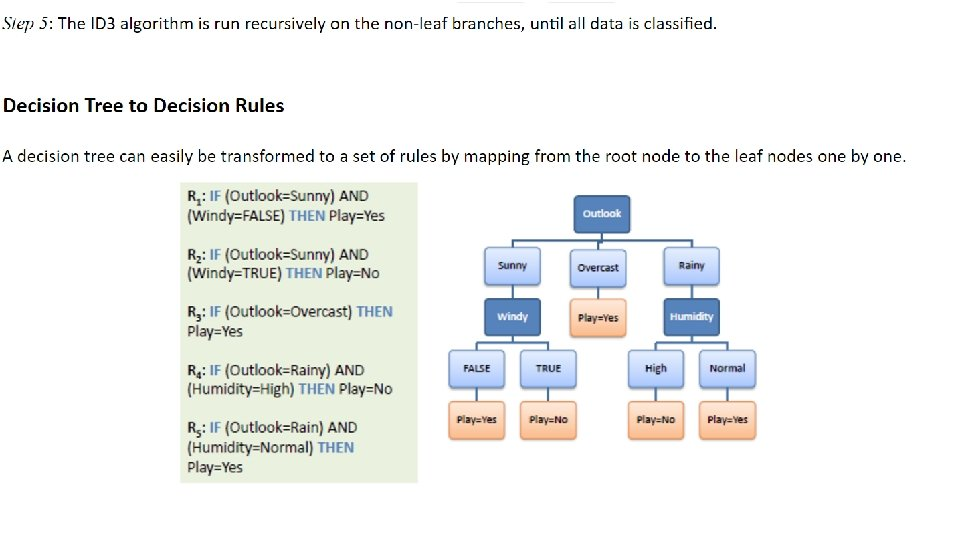# Decision Trees Decision Trees Supervised Learning learn decision

• Slides: 12Decision TreesDecision Trees • Supervised Learning • learn decision tree from training examples • predict classes for novel testing examples • Generalization is how well we do on the testing examples. • Only works if we can learn the underlying structure of the data.Choosing attributes • How do we ﬁnd a decision tree that agrees with the training data? • Could just choose a tree that has one path to a leaf for each example - but this just memorizes the observations (assuming data are consistent) - we want it to generalize to new examples • Ideally, best attribute would partition the data into positive and negative examples • Strategy (greedy): - choose attributes that give the best partition ﬁrst • Want correct classiﬁcation with fewest number of tests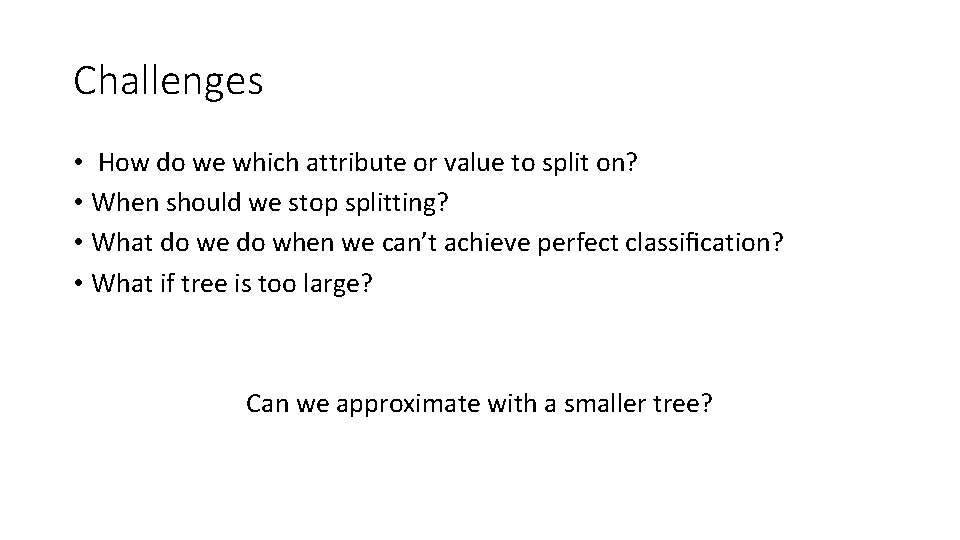Challenges • How do we which attribute or value to split on? • When should we stop splitting? • What do we do when we can’t achieve perfect classiﬁcation? • What if tree is too large? Can we approximate with a smaller tree?Basic algorithm for learning decision trees • start with whole training data • select attribute or value along dimension that gives “best” split • create child nodes based on split • recurse on each child using child data until a stopping criterion is reached • all examples have same class • amount of data is too small • tree too large • Central problem: How do we choose the “best” attribute?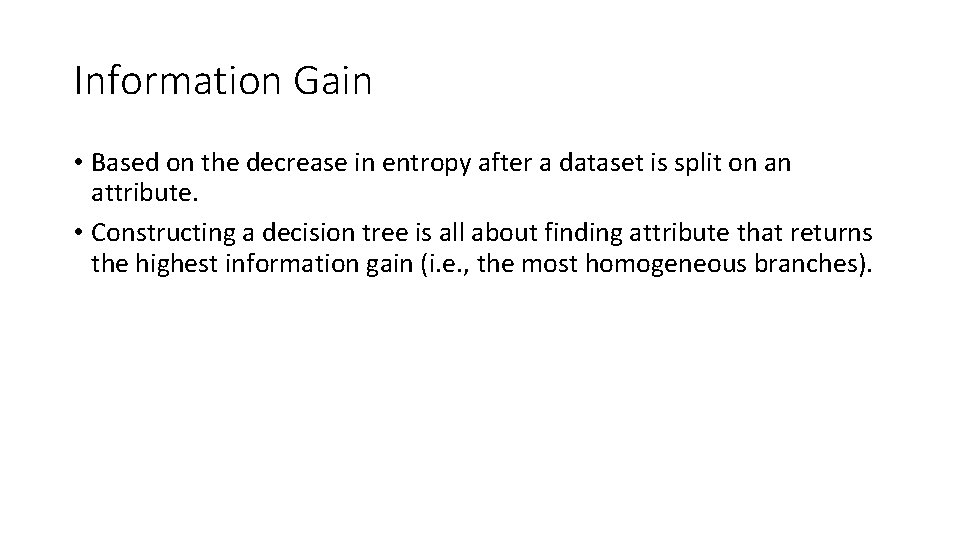Information Gain • Based on the decrease in entropy after a dataset is split on an attribute. • Constructing a decision tree is all about finding attribute that returns the highest information gain (i. e. , the most homogeneous branches).Entropy calculates the homogeneity of a sample If the sample is completely homogeneous the entropy is zero and if the sample is an equally divided it has entropy of one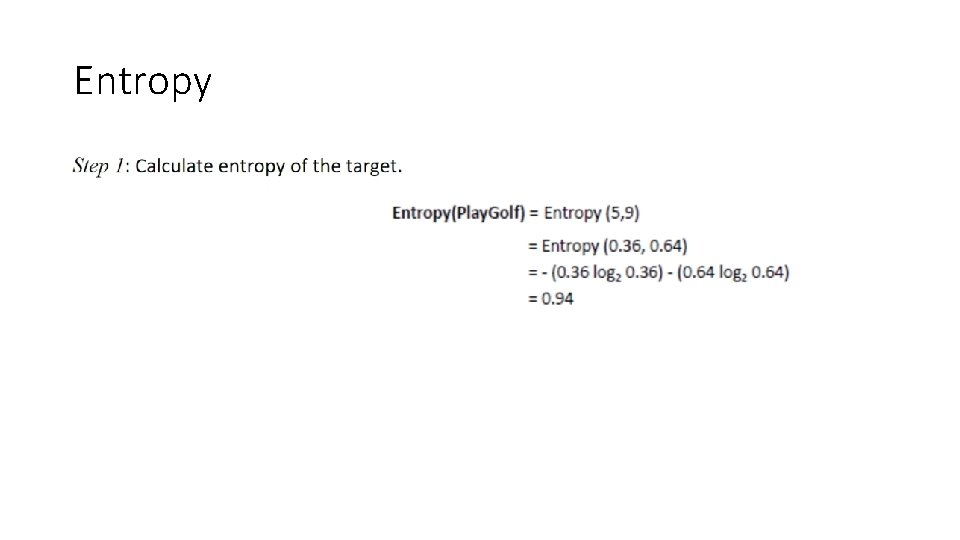Entropy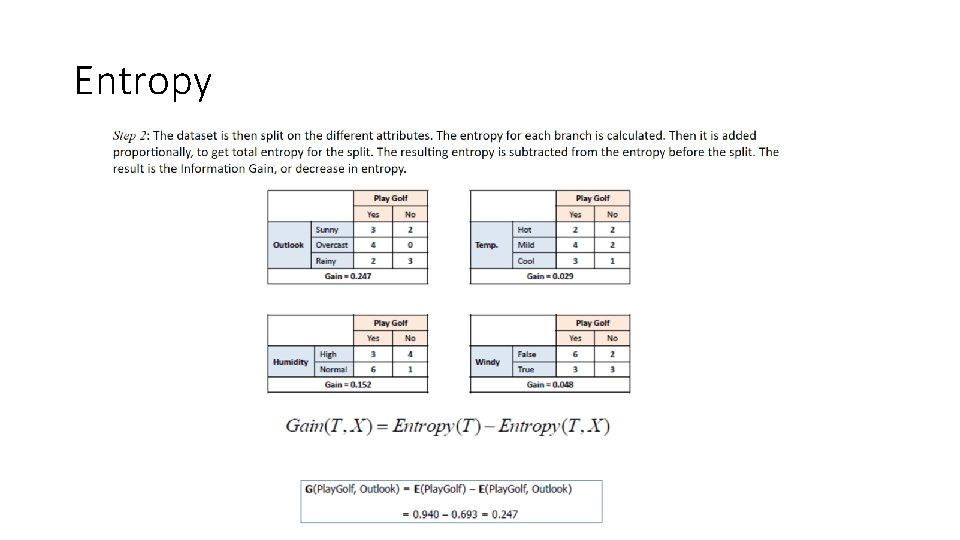Entropy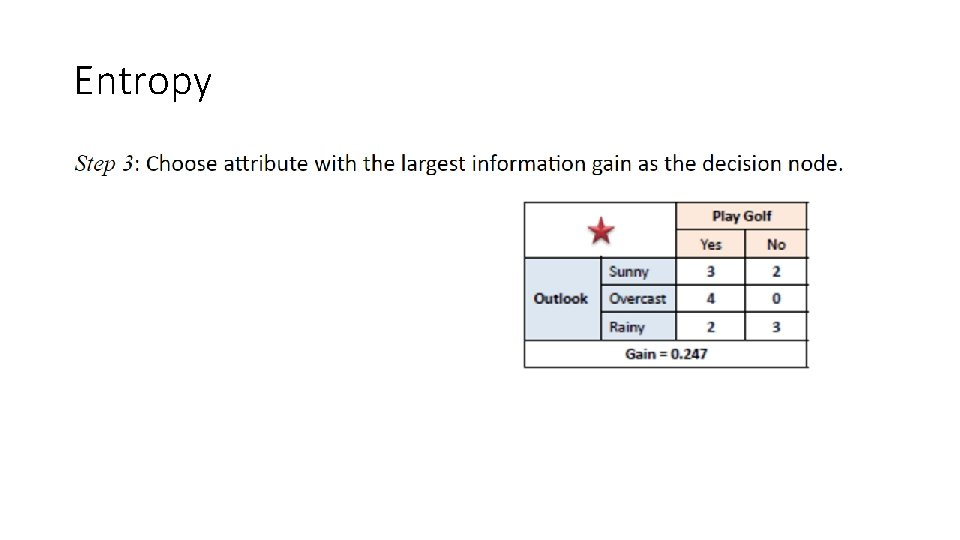Entropy